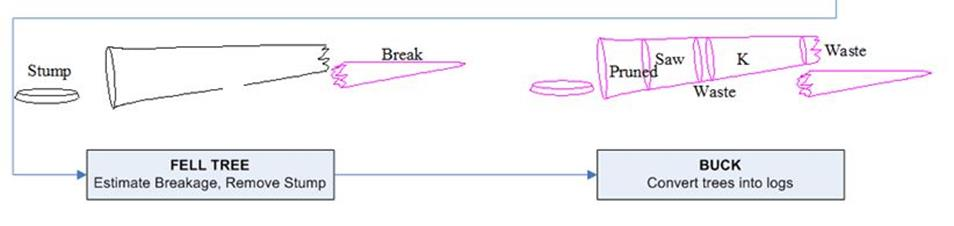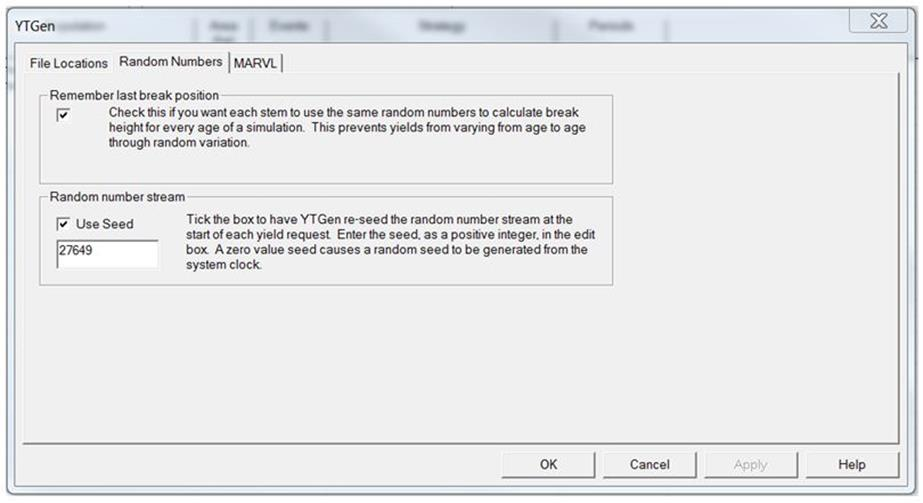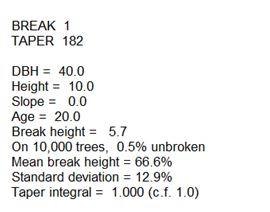+64 7 350 3209 +61 2 8011 3645 info@interpine.co.nz
Select Page

What is random numbers and how are these used within YTGen?

An example use of random numbers in YTGEN is for the calculation of height at which a stem breaks when felled e.g. the whereabouts of break, random numbers hereby only determinate the randomness of a breakage function used. They can also be used in other modelling workflows; such as windthrow or growth modelling.Figure 1 break and converting the tree into logs.

From time to time we are asked, how random numbers work when running the same yield request over and over again and still produces the same result?

To answer this question we have to understand where random number comes from within YTGEN.   Random numbers are controlled within YTGen > Select Options Menu and then Random Numbers tab.Figure 2 Random Number interface

As default the Random Number settings are set as in figure 2, and by so they are not fully random but a long list numbers which is the same for all YTGen users when keeping the start seed of the random number sequence the same (27649). Hereby all users keep the same starting point. The effect of keeping the same seed number is the breakage of each individual tree will remain the same when yield request is run by different users. Default settings are preferable since it enables users to produce the same YTGen result.

What is re-seeding a random number?

In practise, software can’t generate numbers that are truly random. One of the virtues of modern computing is that the same code with the same inputs will produce the same outputs. To circumvent this virtue the pieces of software code that are called upon to produce random numbers use a trick to turn absolutely reproducible numbers into ones that look random. Without going into the details of how they actually do it, the effect can usefully be thought of like this:

1. Take all the integers from 0 through to some very big number (2^32 in the case of YTGen) and put them in a list.

2. Shuffle the list thoroughly, but reproducibly.

3. When asked for a random number, take the first number in the list, then the second and so on.

4. All that “random” means in this case is that there is no correlation between consecutive numbers. It doesn’t mean that we can’t guess what the next number will be.

One practical implication of this approach is if you start at the same position in the list and take numbers in the same order, you will produce the same sequence, or stream, of random numbers. Another implication is you can opt to start at a different position in the list and produce a different, but equally reproducible, stream. “Re-seeding” a random number stream is a term that means “starting at a known position”.

In summary random numbers assigns randomness to the breakage function which will evenly distributes breakage as a function of height, see below figure 3 (scenario; Breakage 1, DBH 40cm, Height 10m and 1.000 stems).Figure 3 breakage parameters

Figure 4 shows the distribution of breakage. A few trees does not break and some are even higher then the projected height of 10 meters. This is due to our normal distribution with a mean of 0.666 and a standard deviation of 0.129 within a 99% confidence interval (z=2.56). On average 99% of values fall between 0.3358-0.9963 times the target height (e.g. 10m). The breaks which occures outside 0.3358-0.9963 is that 1 percent which falls outside our Figure 4 distribution of breakage of 1.000 stems

If you have any questions on related to YTGen please feel free to contact us.